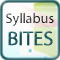# Syllabus bites – revisiting proportion

Secondary KLA:
Mathematics
Educational levels:
Year 7, Year 8This is the first in a series of Syllabus bites related to direct and indirect proportion. Students revise the concept of ratio. They create short visual explanations showing how problems can be solved.

## NSW syllabus outcomes

(MA4-1WM) communicates and connects mathematical ideas using appropriate terminology, diagrams and symbols

(MA4-2WM) applies appropriate mathematical techniques to solve problems

(MA4-3WM) recognises and explains mathematical relationships using reasoning

(MA4-7NA) operates with ratios and rates, and explores their graphical representation

(MA5.2-1WM) selects appropriate notations and conventions to communicate mathematical ideas and solutions

(MA5.2-2WM) interprets mathematical or real-life situations, systematically applying appropriate strategies to solve problems

(MA5.2-5NA) recognises direct and indirect proportion, and solves problems involving direct proportion

## Australian curriculum content descriptions

(ACMNA173) Recognise and solve problems involving simple ratios

(ACMNA188) Solve a range of problems involving rates and ratios, with and without digital technologies

(ACMNA208) Solve problems involving direct proportion. Explore the relationship between graphs and equations corresponding to simple rate problems

Resource type:
Interactive Resource
ScOT topics:
Ratios
File type:
text/html
Language/s:
en-AU
Author:
State of NSW, Department of Education
Publisher:
State of NSW, Department of Education
Date created:
Wednesday, 7 November 2018

Resource ID: d0b08b77-830f-4358-9a05-b5d4d0876b95